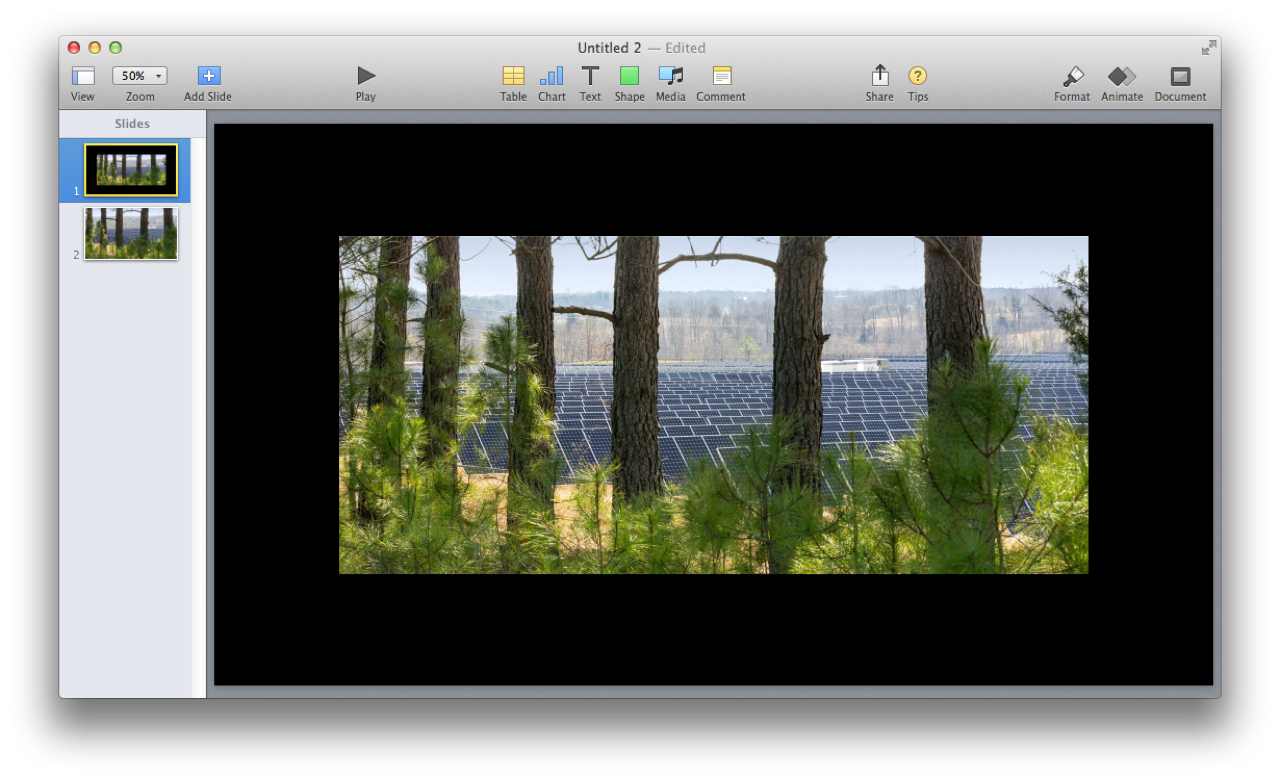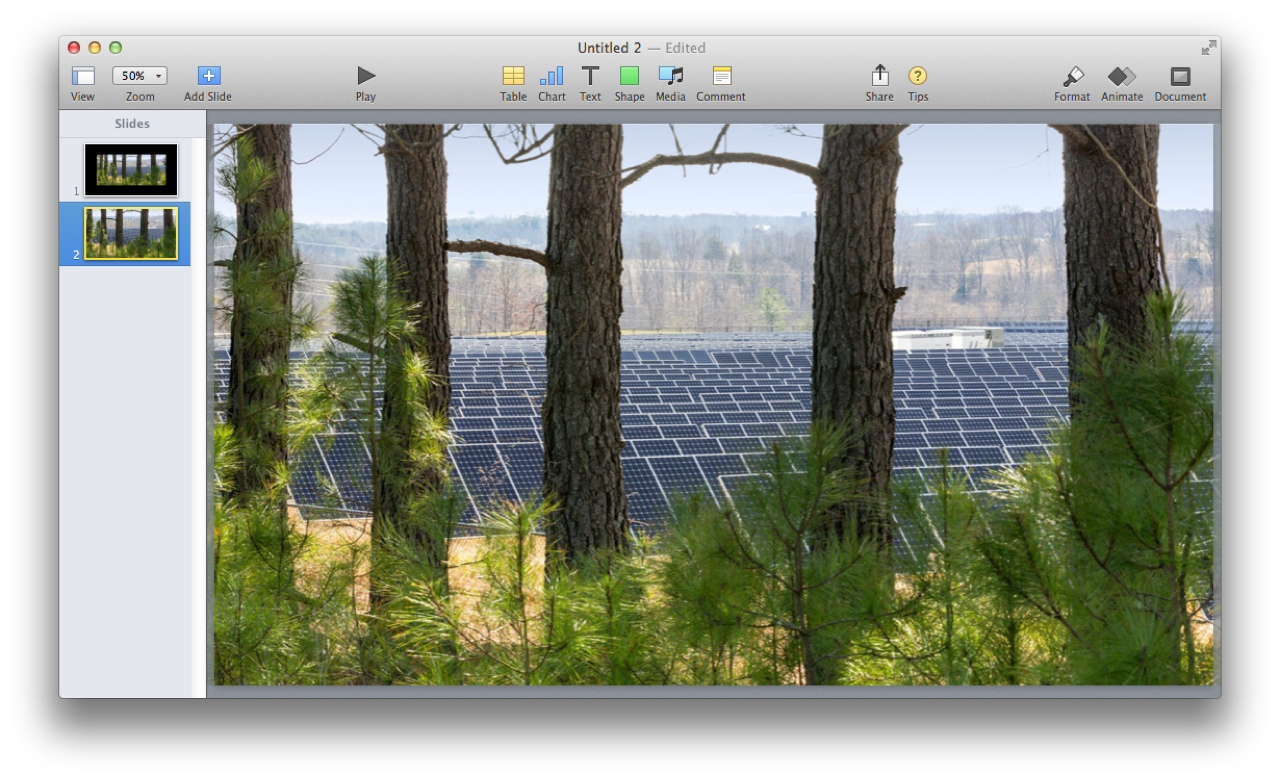Image Slides from Files

Here is a script that demonstrates the technique for creating new image slides from selected image files. You have the option to use the standard image import scaling or sizing the image to fill the slide.(⬆ see above ) Image added to slide using the Keynote application’s default import methodology: if an image dimension is smaller than a corresponding slide dimension, then it is added at normal size; if an image dimension is greater than a corresponding slide dimension, then it is scaled to the corresponding slide dimension. The added image is centered in the window. In the example above, both dimensions of the image are smaller than their corresponding slide dimensions.

(⬇ see below ) Image added to slide using Scale to Fill method: adjust the image as needed (scale up or down) so that all the slide area is covered.